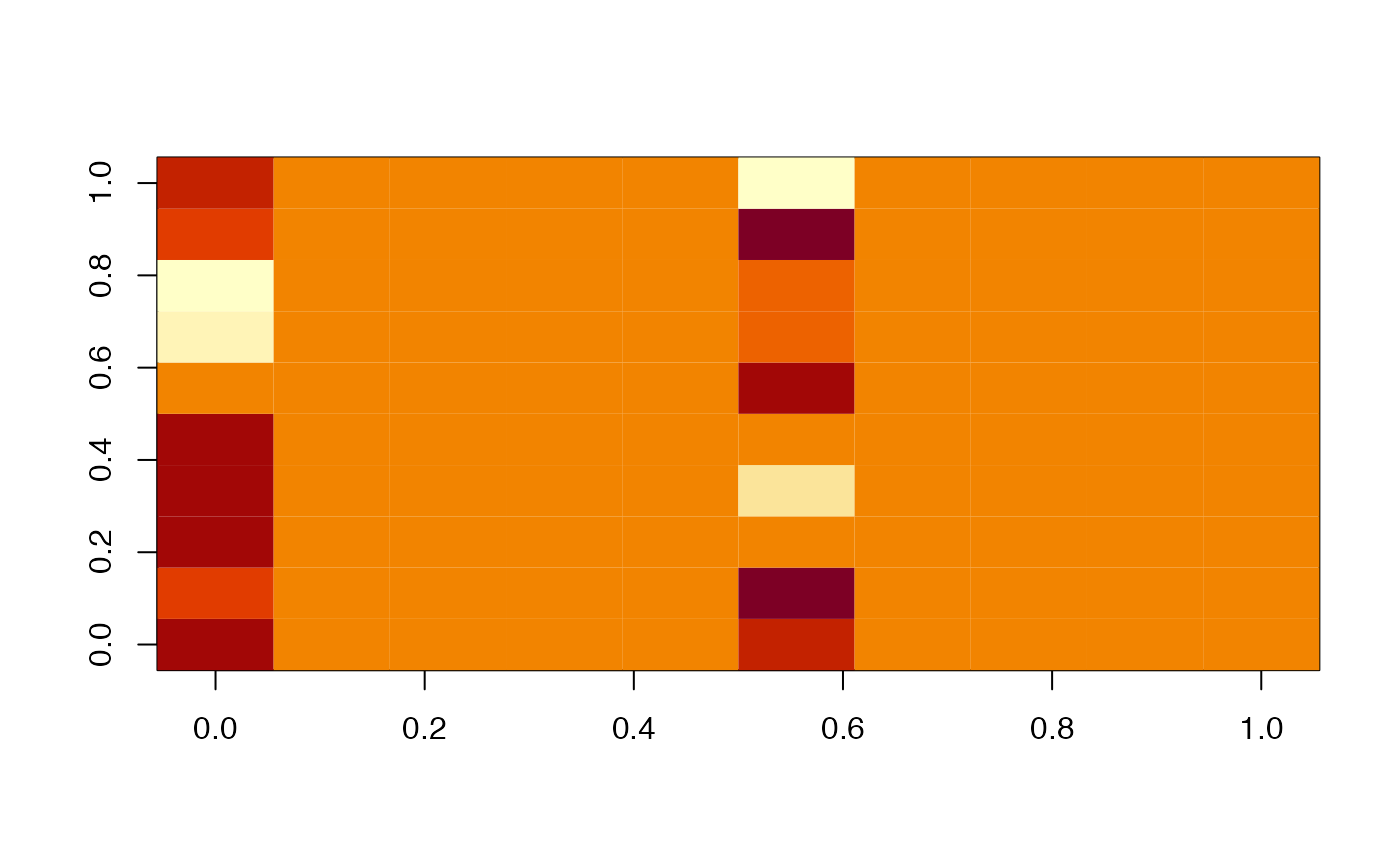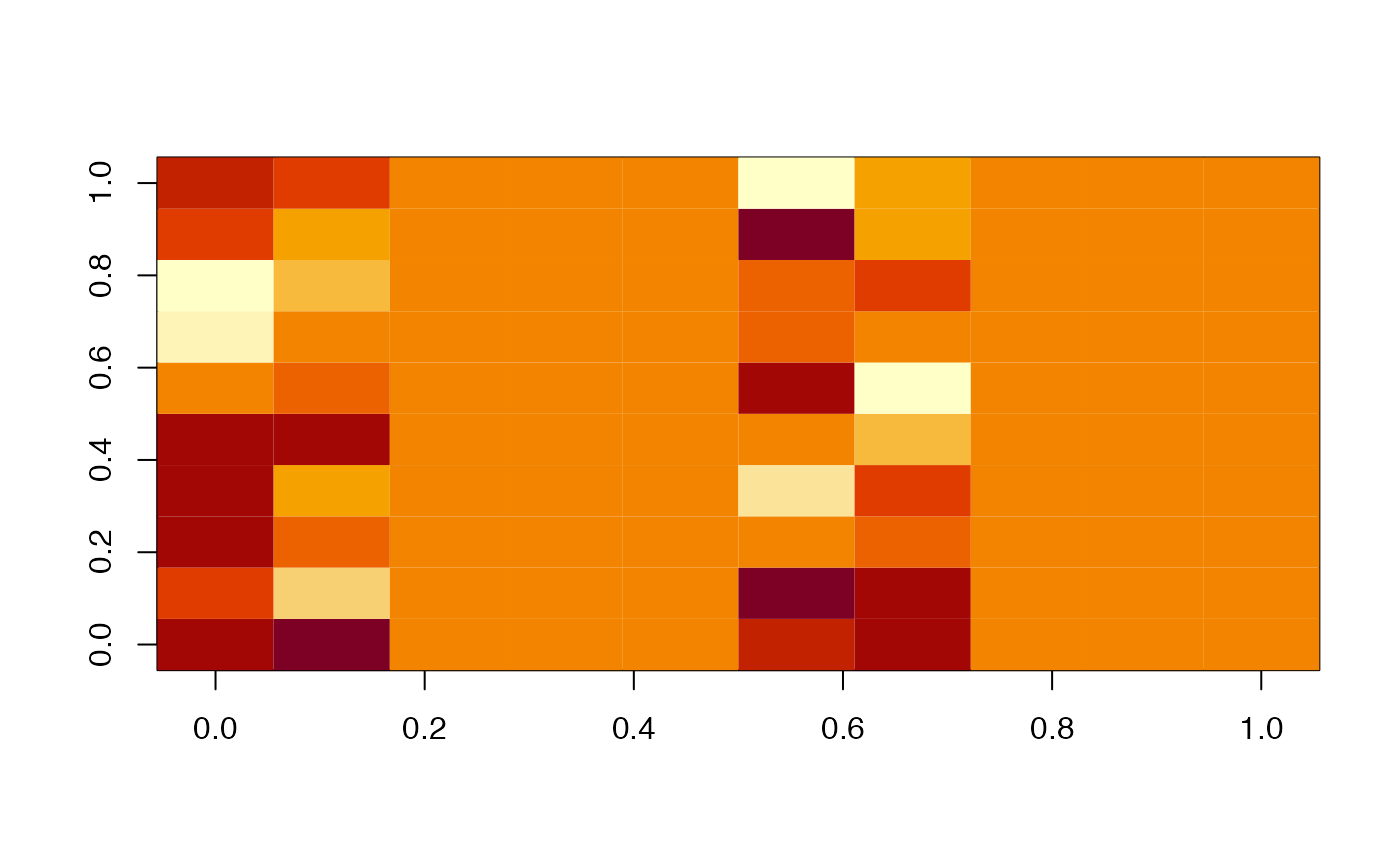Mask an object, typically to produce a copy with some values zeroed out

mask(x, ...)

# S3 method for im3d
invert = FALSE, ...)

## Arguments

x Object to be masked Additional arguments passed to methods An im3d object, an array or a vector with dimensions compatible with x. Optional numeric vector of pixel values or character vector defining named materials. Whether to return an im3d object based on x or just the values from x matching the mask. Whether to invert the voxel selection (default FALSE)

## Value

an object with attributes matching x and elements with value as.vector(TRUE, mode=mode) i.e. TRUE, 1, 0x01 and as.vector(FALSE, mode=mode) i.e. FALSE, 0, 0x00 as appropriate.

A copy of x with

## Details

Note that mask.im3d passes ... arguments on to im3d

Other im3d: as.im3d, boundingbox, im3d-coords, im3d-io, im3d, imexpand.grid, imslice, is.im3d, origin, projection, threshold, unmask, voxdims
x=im3d(array(rnorm(1000),dim=c(10,10,10)), BoundingBox=c(20,200,100,200,200,300))
image(x[,,1])image(mask(x, mask=m, levels=1)[,,1])image(mask(x, mask=m, levels=1:2)[,,1])